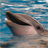# QlikView App Dev

Discussion Board for collaboration related to QlikView App Development.

cancel
Showing results for
Did you mean:Creator II

## What exactly a Fractile function does?

Hi,

Can some one explain me what the Fractile function does?

For example, I used this script,

Country, Value

USA, 12

USA, 14.5

USA, 6.6

USA, 4.5

USA, 7.8

USA, 9.4

UK, 11.3

UK, 10.1

UK, 3.2

UK, 5.6

UK, 3.9

UK, 6.9

];

And in the UI, in the text box, i use the function =Fractile({<Country = {'UK'}>}Value, .75),

I get a value of 9.3.

Using this example, can some one explain what this Fractile function is doing?

1 Solution

Accepted SolutionsCreator II
Author

Here i got the correct Calculation,

UK has 6 values,

UK, 11.3

UK, 10.1

UK, 3.2

UK, 5.6

UK, 3.9

UK, 6.9

So, the total number, "n"=6.

So, if i use the function Fractile(Value, 0.75), it does the following calculation.

(n+1) x 0.75 = 7 x 0.75 = 5.25

As, the decimal value is less than .45, 5.25 considers 5th ('y') value (when the values are arranged ascending order), that is 10.1.

10.1 - ('y value' - 'value above y value')*0.25  --> So, here 10.1- (10.1 - 6.9) x 0.25  = 9.23

This is how the fractile function does the calculation and give the result.

7 RepliesMVP

The qv help itself explains it quite nicely.

 fractile ([{set_expression}] [ distinct ] [ total [] ] expression, fractile) returns the aggregated fractile of expression iterated over the chart dimension(s). The function argument expressions must not contain aggregation functions, unless these inner aggregations contain the total qualifier. If the word total occurs before the function arguments the calculation will be made over all possible values given the current selections but disregarding the chart dimension variables. The total qualifier may be followed by a list of one or more field names within angle brackets. These field names should be a subset of the chart dimension variables. In this case the calculation will be made disregarding all chart dimension variables except those listed, i.e. one value will be returned for each combination of field values in the listed dimension fields. Also fields which are not currently a dimension in a chart may be included in the list. This may be useful in the case of group dimensions, where the dimension fields are not fixed. Listing all of the variables in the group causes the function to work when the cycle or drill-down level changes. Examples: fractile( Sales, 0.75 ) fractile( X*Y/3, 0.9 ) fractile( total Price, 0.25 ) fractile( total Price )

If you have doubt about what fractile is meant for, follow the links that you are redirected to by: Fractile functionMVP

Fractile which is also known as quantile is the total division of something into equivalent subgroups like deciles, quartiles, percentiles, terciles and quintiles. Quantile is defined as a point taken at certain intervals from a random variable's cumulative distribution function (CDF).

More you can read from QliKView Refrence Manual and from below link..

Use of Fractile()Creator II
Author

Hi Tresesco. Thanks for the response. I know that it is in help. But i could not understand it clearly and that is the reason why i gave an example and asked for a explanationEmployee

In your specific example, that means that UK values <9.3 fall in the bottom 75% of all UK valuesMVP

And if you wonder why you get 9.3 though this value is not contained in your data set, well, it's an interpolated value (because you haven't input enough values to really exactely get the 75% threshold).Partner

Hi,

Hope this example helps you.

Just now this code in your qvw file

RowNo() as R,

'A' as Type

AutoGenerate 100;

- Take the two fields in table box.

- Take one st. table and add dim as Type and exp as Fractile(R,.75)

- Now you will find the value as 75 in straight table, fractile will give the result value of the percentage what we had given.

- We have 1 to 100 values, in that 75 is at 75%

Thanks,

JaganCreator II
Author

Here i got the correct Calculation,

UK has 6 values,

UK, 11.3

UK, 10.1

UK, 3.2

UK, 5.6

UK, 3.9

UK, 6.9

So, the total number, "n"=6.

So, if i use the function Fractile(Value, 0.75), it does the following calculation.

(n+1) x 0.75 = 7 x 0.75 = 5.25

As, the decimal value is less than .45, 5.25 considers 5th ('y') value (when the values are arranged ascending order), that is 10.1.

10.1 - ('y value' - 'value above y value')*0.25  --> So, here 10.1- (10.1 - 6.9) x 0.25  = 9.23

This is how the fractile function does the calculation and give the result.